# 前言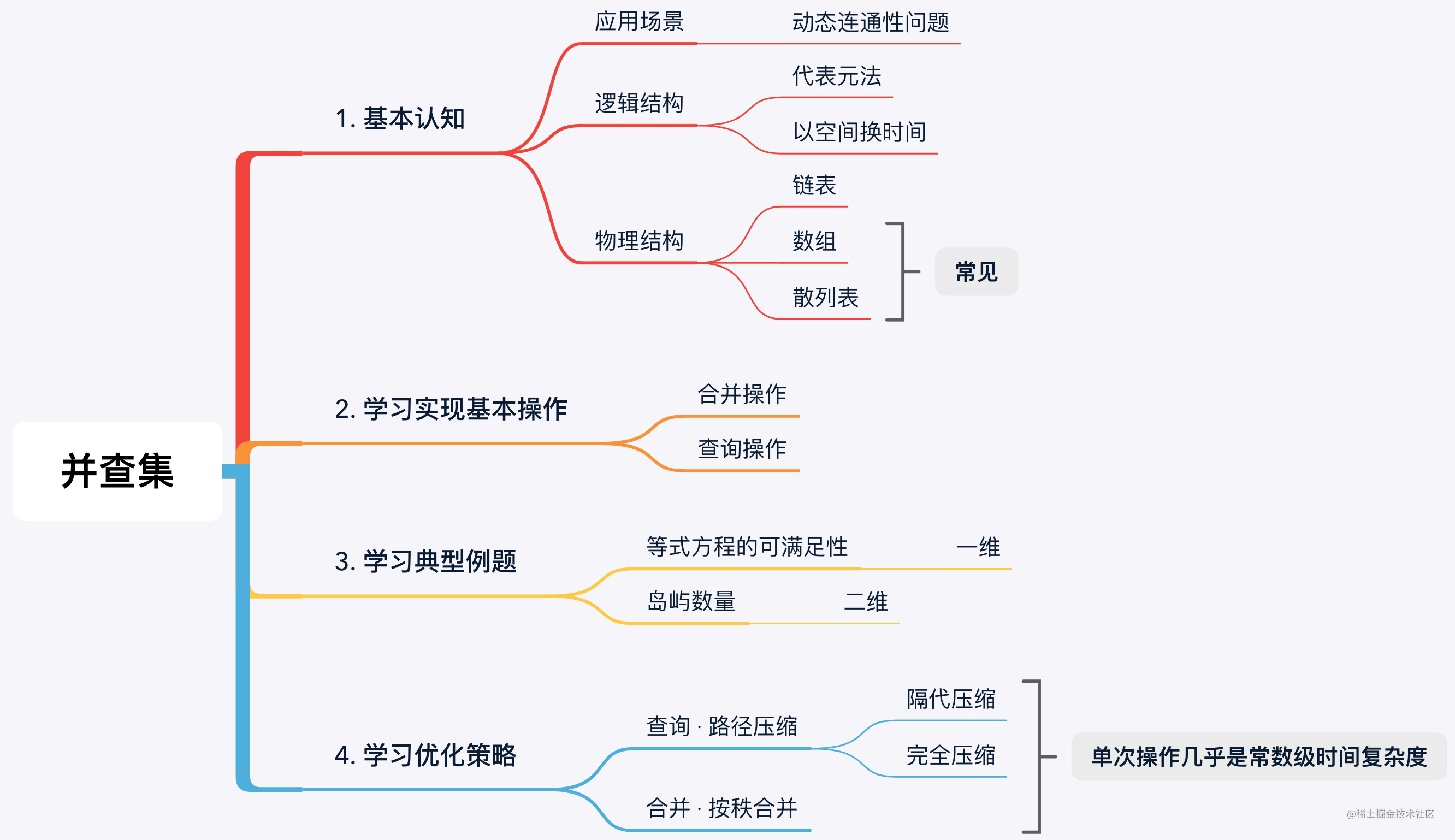# 1. 认识并查集

### 1.1 并查集用于解决什么问题？

`连通问题与路径问题示意图`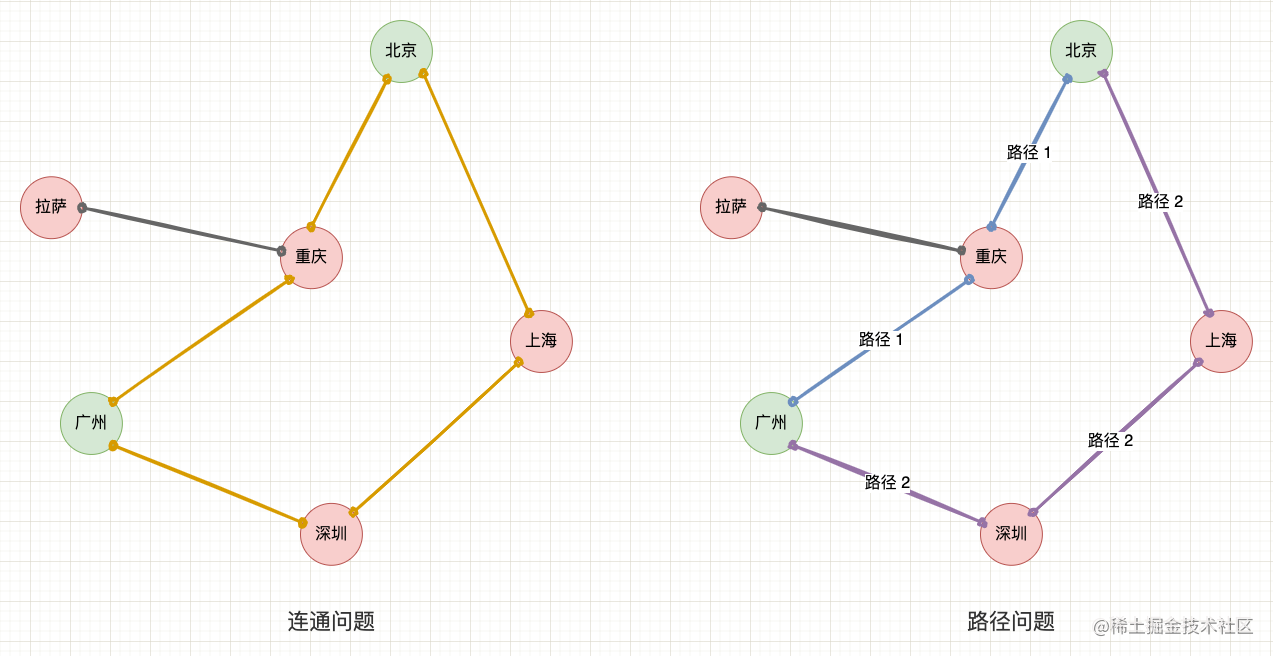`动态连通问题`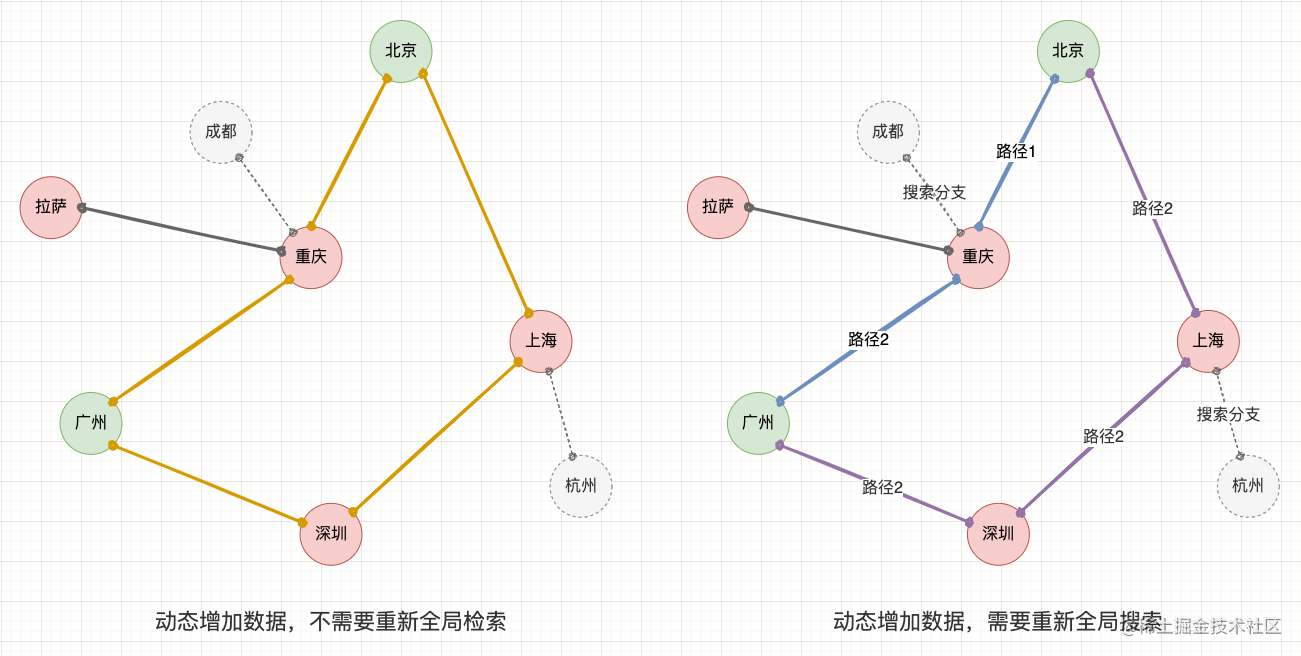### 1.2 并查集的逻辑结构

`并查集的逻辑结构和物理结构`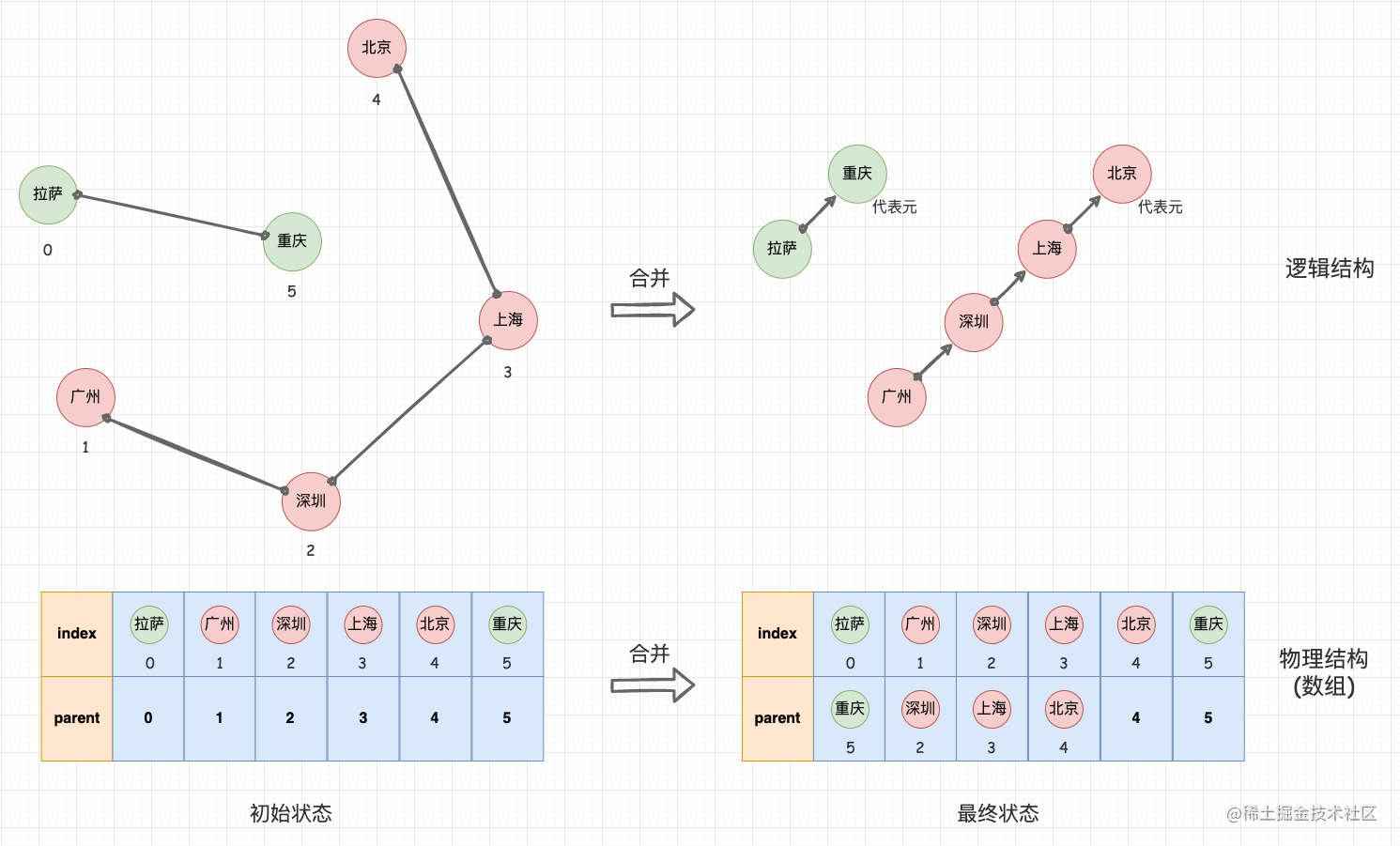### 1.3 并查集的物理结构

• 链表实现： 为每个元素创建一个链表节点，每个节点持有指向父节点的指针，通过指针的的指向关系来构建集合的连接关系，而根节点（代表元）的父节点指针指向节点本身；
• 数组实现： 创建与元素个数相同大小的数组，每个数组下标与每个元素一一对应，数组的值表示父节点的下标位置，而根节点（代表元）所处位置的值就是数组下标，表示指向本身。

`基于数组的并查集`

```kotlin // 数组实现适合元素个数固定的场景 class UnionFind(n: Int) { // 创建一个长度为 n 的数组，每个位置上的值初始化数组下标，表示初始化时有 n 个子集 private val parent = IntArray(n) { it } ... }```

`基于散列表的并查集`

```kotlin // 散列表实现适合元素个数不固定的场景 class UnionFind() { // 创建一个空散列表， private val parent = HashMap()

``````// 查询操作
fun find(x: Int): Int {
// 1. parent[x] 为 null 表示首次查询，先加入散列表中并指向自身
if (null == parent[x]) {
parent[x] = x
return x
}
// 下文说明查询操作细节...
}
``````

} ```

# 2. 并查集的基本概念

### 2.1 合并操作与查询操作

“并查集，并查集”，顾名思义并查集就是由 “并” 和 “查” 这两个最基本的操作组成的：

• Find 查询操作： 沿着只用链条找到根节点（代表元）。如果两个元素的根节点相同，则说明两个元素是否属于同一个子集，否则属于不同自己；
• Union 合并操作： 将两个元素的根节点合并，也表示将两个子集合并为一个子集。

`基于数组的并查集`

```kotlin class UnionFind(n: Int) {

``````// 创建一个长度为 n 的数组，每个位置上的值初始化数组下标，表示初始化时有 n 个子集
val parent = IntArray(n) { it }

// 查询操作（遍历写法）
fun find(x: Int): Int {
var key = x
while (key != parent[key]) {
key = parent[key]
}
return key
}

// 合并操作
fun union(x: Int, y: Int) {
// 1. 分别找出两个元素的根节点
val rootX = find(x)
val rootY = find(y)
// 2. 任意选择其中一个根节点成为另一个根节点的子树
parent[rootY] = rootX
}

// 判断连通性
fun isConnected(x: Int, y: Int): Boolean {
// 判断根节点是否相同
return find(x) == find(y)
}

// 查询操作（递归写法）
fun find(x: Int): Int {
var key = x
if (key != parent[key]) {
return find(parent[key])
}
return key
}
``````

} ```

`合并与查询示意图`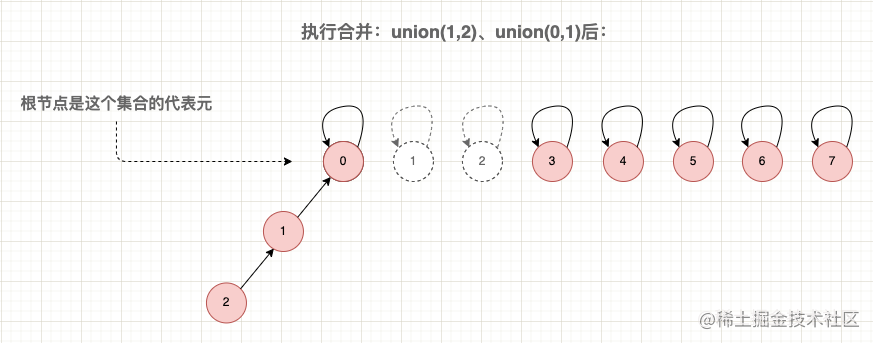### 2.2 连通分量

```kotlin class UnionFind(n: Int) {

``````private val parent = IntArray(n) { it }

// 连通分量计数，初始值为元素个数 n
var count = n

// 合并操作
fun union(x: Int, y: Int) {
val rootX = find(x)
val rootY = find(y)
if(rootX == rootY){
// 未发生合并，则不需要减一
return
}
// 合并后，连通分量减一
parent[rootY] = rootX
count --
}
...
``````

} ```

`连通分量示意图`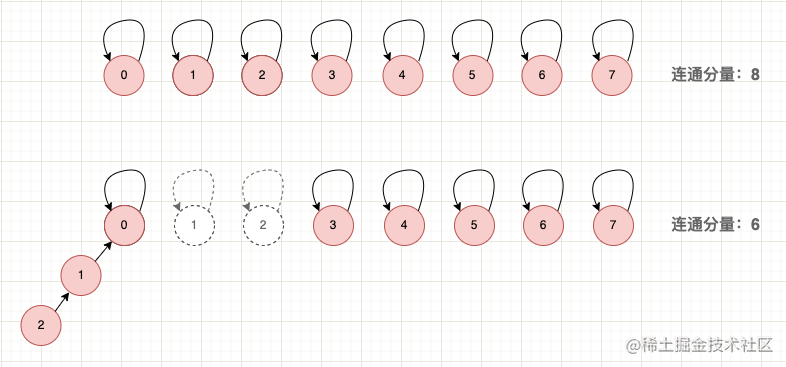# 3. 典型例题 · 等式方程的可满足性

`LeetCode 例题`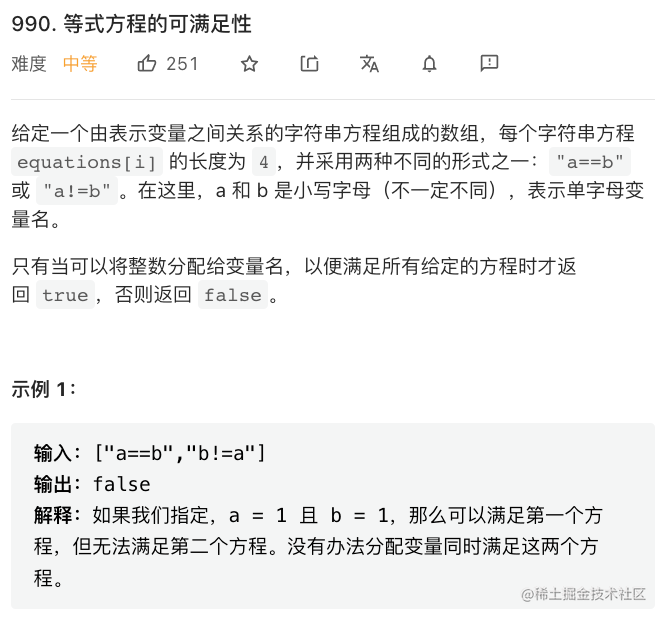• 1、先遍历所有等式，将等式中的两个变量合并到同一个子集中，最终构造一个并查集；
• 2、再遍历所有不等式，判断不等式中的两个变量是否处于同一个子集。是则说明有冲突，即等式方程不成立。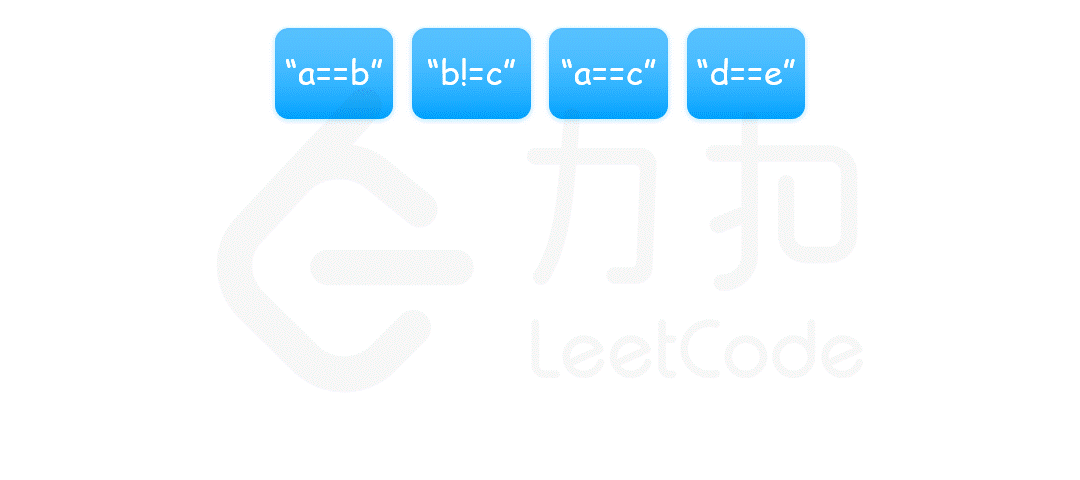—— 图片引用自 LeetCode 官方题解

`题解`

```kotlin // 未优化版本 class Solution { fun equationsPossible(equations: Array): Boolean { // 26 个小写字母的并查集 val unionFind = UnionFind(26)

``````    // 合并所有等式
for (equation in equations.filter { it == '=' }) {
unionFind.union(equation.first(), equation.second())
}
// 检查不等式是否与连通性冲突
for (equation in equations.filter { it == '!' }) {
if (unionFind.isConnected(equation.first(), equation.second())) {
return false
}
}
return true
}

private fun String.first(): Int {
return this.toInt() - 97
}

private fun String.second(): Int {
return this.toInt() - 97
}

private class UnionFind() {
// 代码略
}
``````

} ```

# 4. 并查集的优化

### 4.1 父节点重要吗？

`父节点并不重要`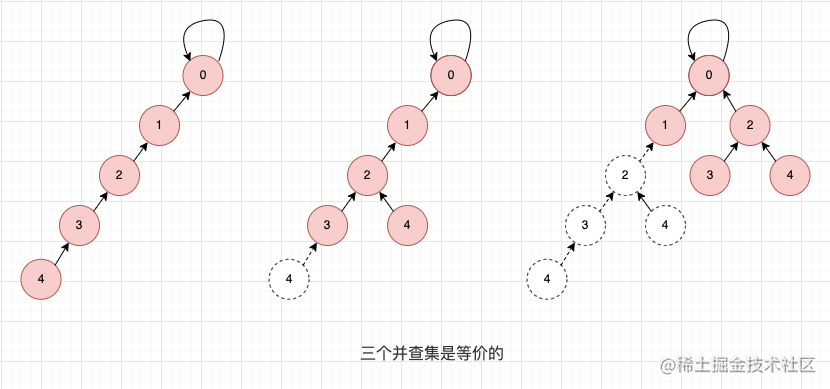### 4.2 路径压缩（Path Compression）

• 隔代压缩： 调整父节点的指向，使其指向父节点的父节点；
• 完全压缩： 调整父节点的指向，使其直接指向根节点。

`路径压缩示意图`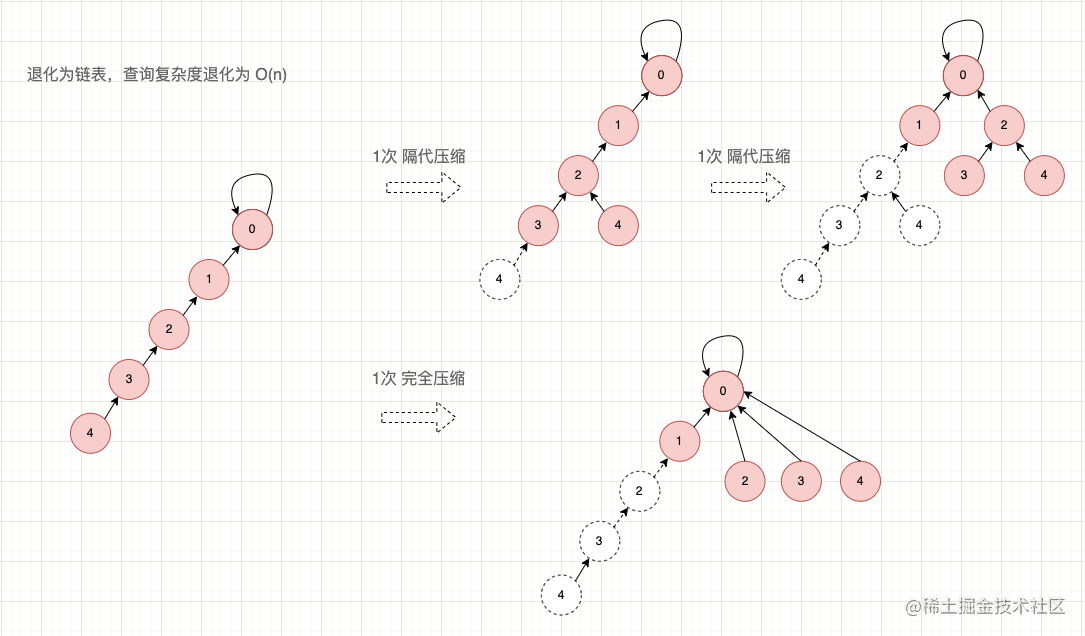`路径压缩示例程序`

```kotlin // 遍历写法 fun find(x: Int): Int { var key = x while (key != parent[key]) { parent[key] = parent[parent[key]] key = parent[key] } return key }

// 递归写法 fun find(x: Int): Int { var key = x if (key != parent[key]) { parent[key] = find(parent[key]) return parent[key] } return key } ```

### 4.3 按秩合并（Union by Rank）

`按秩合并示意图`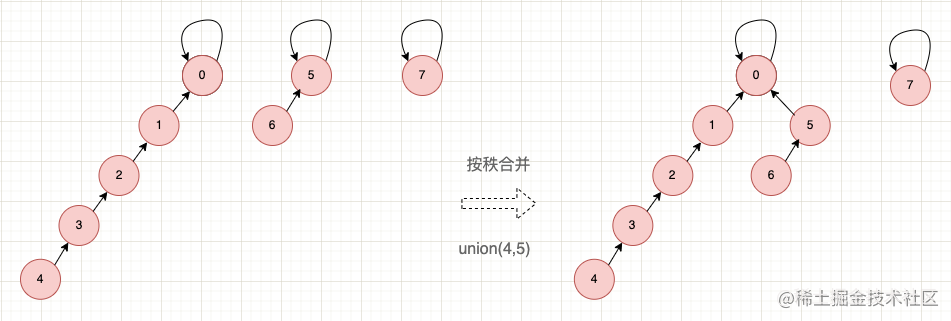`按秩合并示例程序`

```kotlin private class UnionFind(n: Int) { // 父节点 private val parent = IntArray(n) { it }

``````// 节点的高度
private val rank = IntArray(n) { 1 }

// 连通分量
var count = n
private set

// 查询（路径压缩）
fun find(x: Int): Int {
var key = x
while (key != parent[key]) {
parent[key] = parent[parent[key]]
key = parent[key]
}
return key
}

// 合并（按秩合并）
fun union(key1: Int, key2: Int) {
val root1 = find(key1)
val root2 = find(key2)

if (root1 == root2) {
return
}
if (rank[root1] > rank[root2]) {
// root1 的高度更大，让 root2 成为子树，树的高度不变
parent[root2] = root1
} else if (rank[root2] > rank[root1]) {
// root2 的高度更大，让 root1 成为子树，树的高度不变
parent[root1] = root2
} else {
// 高度相同，谁当子树都一样
parent[root1] = root2
// root2 的高度加一
rank[root2]++
//  或
//  parent[root2] = root1
//  rank[root1] ++
}
count--
}
``````

} ```

# 5. 典型例题 · 岛屿数量（二维）

`LeetCode 例题`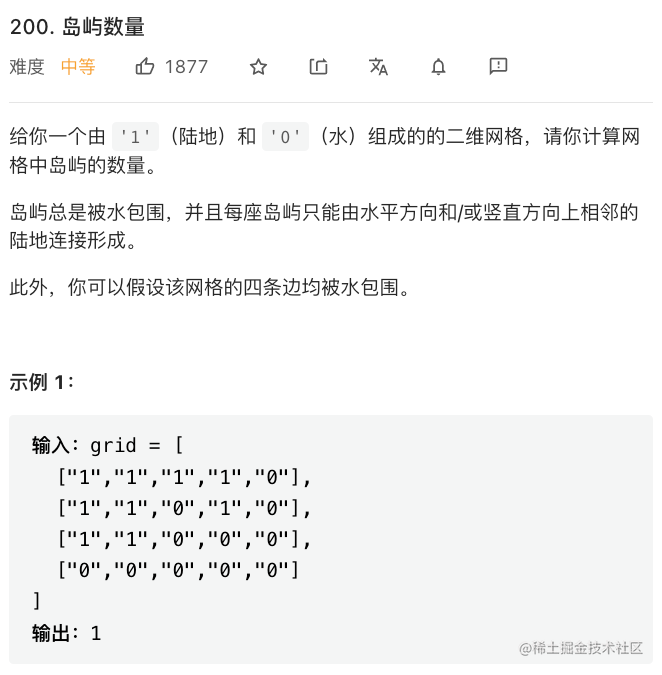`并查集解法`

```kotlin class Solution { fun numIslands(grid: Array): Int {

``````    // 位置
fun position(row: Int, column: Int) = row * grid.size + column

// 并查集
val unionFind = UnionFind(grid)

// 偏移量数组（向右和向下）
val directions = arrayOf(intArrayOf(0, 1), intArrayOf(1, 0))

// 边界检查
fun checkBound(row: Int, column: Int): Boolean {
return (row in grid.indices) and (column in grid.indices)
}

for (row in grid.indices) {
for (column in grid.indices) {
if ('1' == grid[row][column]) {
// 消费（避免后续的遍历中重复搜索）
grid[row][column] = '0'
for (direction in directions) {
val newRow = row + direction
val newColumn = column + direction
if (checkBound(newRow, newColumn) && '1' == grid[newRow][newColumn]) {
unionFind.union(position(newRow, newColumn), position(row, column))
}
}
}
}
}
return unionFind.count
}

private class UnionFind(grid: Array<CharArray>) {

// 父节点
private val parent = IntArray(grid.size * grid.size) { it }

// 节点高度
private val rank = IntArray(grid.size * grid.size) { 1 }

// 连通分量（取格子 1 的总数）
var count = grid.let {
var countOf1 = 0
for (row in grid.indices) {
for (column in grid.indices) {
if ('1' == grid[row][column]) countOf1++
}
}
countOf1
}
private set

// 合并（按秩合并）
fun union(key1: Int, key2: Int) {
val root1 = find(key1)
val root2 = find(key2)
if (root1 == root2) {
// 未发生合并，则不需要减一
return
}
if (rank[root1] > rank[root2]) {
parent[root2] = root1
} else if (rank[root2] > rank[root1]) {
parent[root1] = root2
} else {
parent[root1] = root2
rank[root2]++
}
// 合并后，连通分量减一
count--
}

// 查询（使用路径压缩）
fun find(x: Int): Int {
var key = x
while (key != parent[key]) {
parent[key] = parent[parent[key]]
key = parent[key]
}
return key
}
}
``````

} ```

# 6. 总结

| 并查集 | 题解 | | --- | --- | | 990. 等式方程的可满足性 | 【题解】 | | 200. 岛屿数量 | 【题解】 | | 547. 省份数量 | 【题解】 | | 684. 冗余连接 | 【题解】 | | 685. 冗余连接 II | | | 1319. 连通网络的操作次数 | 【题解】 | | 399. 除法求值 | | | 952. 按公因数计算最大组件大小 | | | 130. 被围绕的区域 | | | 128. 最长连续序列 | | | 721. 账户合并 | | | 765. 情侣牵手 | |

### 参考资料

• 数据结构与算法分析 · Java 语言描述（第 8 章 · 不相互交集类）—— [美] Mark Allen Weiss 著
• 算法导论（第 21 章 · 用于不相交集合的数据结构）—— [美] Thomas H. Cormen 等 著
• 专题 · 并查集 —— LeetCode 出品
• 题目 · 等式方程的可满足性 —— LeetCode 出品